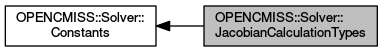OpenCMISS-Iron FORTRAN API Documentation
OPENCMISS::Solver::JacobianCalculationTypes

The Jacobian calculation types for a nonlinear solver. More...

Collaboration diagram for OPENCMISS::Solver::JacobianCalculationTypes:Variables

integer(intg), parameter, public opencmiss_iron::cmfe_solver_newton_jacobian_not_calculated = SOLVER_NEWTON_JACOBIAN_NOT_CALCULATED
The Jacobian values will not be calculated for the nonlinear equations set. More...

integer(intg), parameter, public opencmiss_iron::cmfe_solver_newton_jacobian_equations_calculated = SOLVER_NEWTON_JACOBIAN_EQUATIONS_CALCULATED
The Jacobian values will be calculated analytically for the nonlinear equations set. More...

integer(intg), parameter, public opencmiss_iron::cmfe_solver_newton_jacobian_fd_calculated = SOLVER_NEWTON_JACOBIAN_FD_CALCULATED
The Jacobian values will be calcualted using finite differences for the nonlinear equations set. More...

Detailed Description

The Jacobian calculation types for a nonlinear solver.

OPENCMISS::Solver::Constants,OPENCMISS

Variable Documentation

 integer(intg), parameter, public opencmiss_iron::cmfe_solver_newton_jacobian_equations_calculated = SOLVER_NEWTON_JACOBIAN_EQUATIONS_CALCULATED

The Jacobian values will be calculated analytically for the nonlinear equations set.

OPENCMISS::Solver::JacobianCalculationTypes,OPENCMISS

Definition at line 6143 of file opencmiss_iron.f90.

 integer(intg), parameter, public opencmiss_iron::cmfe_solver_newton_jacobian_fd_calculated = SOLVER_NEWTON_JACOBIAN_FD_CALCULATED

The Jacobian values will be calcualted using finite differences for the nonlinear equations set.